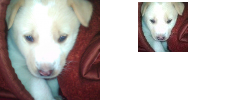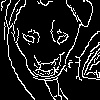# Image Processing in Python (Scaling, Rotating, Shifting and Edge Detection)

• Difficulty Level : Easy
• Last Updated : 17 Sep, 2018

Taking pictures is just a matter of click so why playing around with it should be more than few lines of code. Seems not a case with python. There are quite a few good libraries available in python to process images such as open-cv, Pillow etc. In this article we’ll be using Open CV, an open source library for computer vision. It has C++, python and java interfaces available. It’s highly optimized (written in C/C++) for real time applications in the domain of computer vision.

Let’s start with a simple one i.e Scaling an image.

Scaling an Image :-

Scaling operation increases/reduces size of an image.

 `import` `cv2 ` `import` `numpy as np ` ` `  `FILE_NAME ``=` `'volleyball.jpg'` `try``: ` `    ``# Read image from disk. ` `    ``img ``=` `cv2.imread(FILE_NAME) ` ` `  `    ``# Get number of pixel horizontally and vertically. ` `    ``(height, width) ``=` `img.shape[:``2``] ` ` `  `    ``# Specify the size of image along with interploation methods. ` `    ``# cv2.INTER_AREA is used for shrinking, whereas cv2.INTER_CUBIC ` `    ``# is used for zooming. ` `    ``res ``=` `cv2.resize(img, (``int``(width ``/` `2``), ``int``(height ``/` `2``)), interpolation ``=` `cv2.INTER_CUBIC) ` ` `  `    ``# Write image back to disk. ` `    ``cv2.imwrite(``'result.jpg'``, res) ` ` `  `except` `IOError: ` `    ``print` `(``'Error while reading files !!!'``) `

Output:Rotating an image :-
Images can be rotated to any degree clockwise or otherwise. We just need to define rotation matrix listing rotation point, degree of rotation and the scaling factor.

 `import` `cv2 ` `import` `numpy as np ` ` `  `FILE_NAME ``=` `'volleyball.jpg'` `try``: ` `    ``# Read image from the disk. ` `    ``img ``=` `cv2.imread(FILE_NAME) ` ` `  `    ``# Shape of image in terms of pixels. ` `    ``(rows, cols) ``=` `img.shape[:``2``] ` ` `  `    ``# getRotationMatrix2D creates a matrix needed for transformation. ` `    ``# We want matrix for rotation w.r.t center to 45 degree without scaling. ` `    ``M ``=` `cv2.getRotationMatrix2D((cols ``/` `2``, rows ``/` `2``), ``45``, ``1``) ` `    ``res ``=` `cv2.warpAffine(img, M, (cols, rows)) ` ` `  `    ``# Write image back to disk. ` `    ``cv2.imwrite(``'result.jpg'``, res) ` `except` `IOError: ` `    ``print` `(``'Error while reading files !!!'``) `

Output:Translating an Image :-
Translating an image means shifting it within a given frame of reference.

 `import` `cv2 ` `import` `numpy as np ` ` `  `FILE_NAME ``=` `'volleyball.jpg'` `# Create translation matrix. ` `# If the shift is (x, y) then matrix would be ` `# M = [1 0 x] ` `#     [0 1 y] ` `# Let's shift by (100, 50). ` `M ``=` `np.float32([[``1``, ``0``, ``100``], [``0``, ``1``, ``50``]]) ` ` `  `try``: ` ` `  `    ``# Read image from disk. ` `    ``img ``=` `cv2.imread(FILE_NAME) ` `    ``(rows, cols) ``=` `img.shape[:``2``] ` ` `  `    ``# warpAffine does appropriate shifting given the ` `    ``# translation matrix. ` `    ``res ``=` `cv2.warpAffine(img, M, (cols, rows)) ` ` `  `    ``# Write image back to disk. ` `    ``cv2.imwrite(``'result.jpg'``, res) ` ` `  `except` `IOError: ` `    ``print` `(``'Error while reading files !!!'``) `

Output:Edge detection in an Image :-
The process of image detection involves detecting sharp edges in the image. This edge detection is essential in context of image recognition or object localization/detection. There are several algorithms for detecting edges due to it’s wide applicability. We’ll be using one such algorithm known as Canny Edge Detection.

 `import` `cv2 ` `import` `numpy as np ` ` `  `FILE_NAME ``=` `'volleyball.jpg'` `try``: ` `    ``# Read image from disk. ` `    ``img ``=` `cv2.imread(FILE_NAME) ` ` `  `    ``# Canny edge detection. ` `    ``edges ``=` `cv2.Canny(img, ``100``, ``200``) ` ` `  `    ``# Write image back to disk. ` `    ``cv2.imwrite(``'result.jpg'``, edges) ` `except` `IOError: ` `    ``print` `(``'Error while reading files !!!'``) `

Output:Please refer Github for more details.

My Personal Notes arrow_drop_up
Recommended Articles
Page :